# MCQs on Chemical Equilibrium

Everything tries to achieve minimum energy to remain stable. In a chemical reaction, reactants undergo changes to lower the energy and the reaction proceeds until a low energy state is reached. In a state of chemical equilibrium, the concentration of reactants and products doesn’t change with time and there is no observable change in the system. In a dynamic equilibrium state, the rate of the forward reaction is equal to the rate of backward reaction.

1. Find the pH of a solution when 0.01 M HCl and 0.1 M NaOH are mixed in equal volumes

(a) 12.65

(b) 1.04

(c) 7.0

(d) 2.0

2. Which of the following aqueous solution will be the best conductor of electricity?

(a) NH3

(b) CH3COOH

(c) HCl

(d) C6H12O6

3. In 0.10 M aqueous solution of pyridine (C5H5N), find the percentage of pyridine that forms pyridinium ion (C5H5N+H) (Kb for C5H5N = 1.7 x 10-9 )

(a) 1.6%

(b) 0.77%

(c) 0.0060%

(d) 0.013%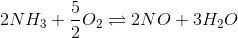4. Find the equilibrium constant of the reaction

If the equilibrium constant for the following reactions are given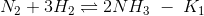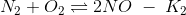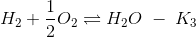(a) K2K3/K1

(b) K1K33/K2

(c) K2K33/K1

(d) K23K3/K1

5. Highest pH will be recorded for which of the following solutions if they are equimolar

(a) AlCl3

(b) BaCl2

(c) BeCl2

(d) LiCl

6. The equilibrium constant is 278 for the reaction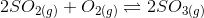at the same temperature, what will be the equilibrium constant for the following reaction?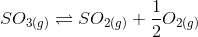(a) 6 x 10-2

(b) 1.8 x 10-3

(c) 1.3 x 10-5

(d) 3.6 x 10-5

7. What will be the pH of a buffer solution having an equal concentration of B and HB (Kb = 10-10 for B)

(a) 7

(b) 4

(c) 10

(d) 6

8. Find the increase in equilibrium concentration of Fe3+ ions if OH ions concentration decreases to 1/4th in the following reaction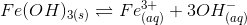(a) 8 times

(b) 16 times

(c) 4 times

(d) 64 times

9. On increasing the concentration of reactants in a reversible reaction, then equilibrium constant will

(a) depend on the concentration

(b) increase

(c) unchanged

(d) decrease

10. Find the conjugate acid of NH2

(a) NH3

(b) NH4OH

(c) NH4+

(d) NH2

## Recommended Videos

#### Introduction to Chemical Equilibrium#### Equilibrium – Top 10 Questions#### Chemical Equilibrium – Le Chatelier’s Principle#### 1 Comment

1. shilpa

it is very useful